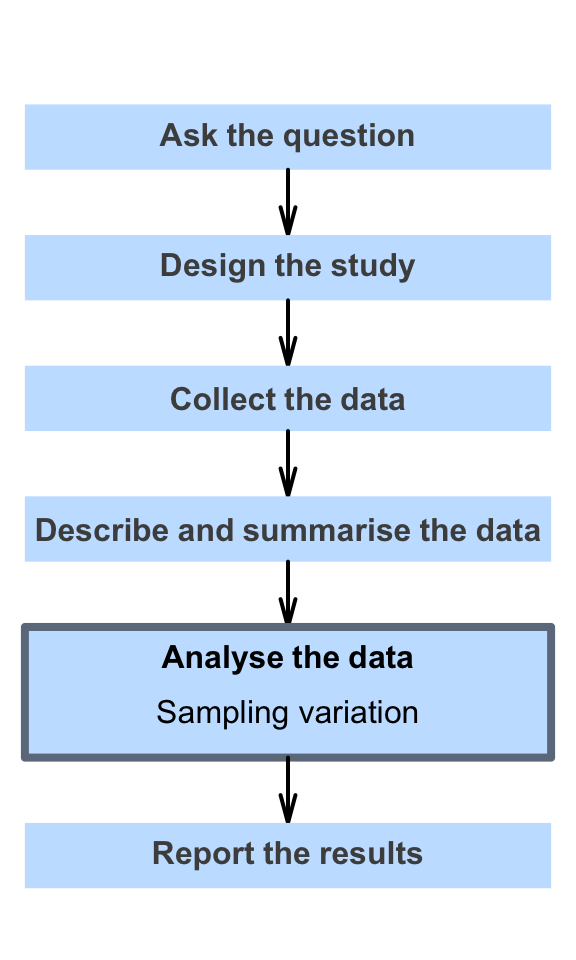# 18 Sampling variation

So far, you have learnt to ask a RQ, identify different ways of obtaining data, design the study, collect the data describe the data, summarise data graphically and numerically, and understand the decision-making process.

In this chapter, you will learn to describe sampling variation. You will learn to:

• explain what a sampling distribution describes.
• explain the difference between variation between individuals and variation in sample statistics.
• determine when a standard error is appropriate to use.
• explain the difference between standard errors and standard deviations.